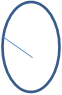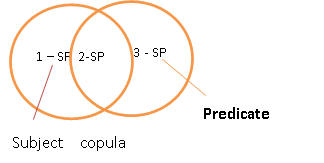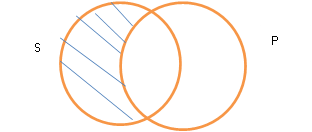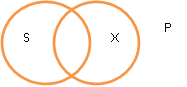# symbolising and diagramming propositions

#### SYMBOLISING AND DAIGRAMMING PROPOSITIONS

Symbolising the A, E, I and O propositions

Each categorical proposition has two terms namely the subject and the predicate terms. These terms can be symbolised using the S and the P respectively.

To assert that “S” has no members, we write an equal sign between S and O in which the O sign represents a null class. Hence to S=0 implies that S has no members. To deny that S has no members, it is represented by

The E proposition asserts that no members of the class S are members of the class P so the product of the product of the two classes is 0 symbolised by the equation SP=0

The I proposition asserts that some members of the subject class are also members of the predicate class “P” and is symbolised by the equation SP≠0.

To symbolise the A and the O proposition, a new method of representing class complement is introduced. For example the complement of “teachers” are “all things which are not students” I.e. the class of all non-students where the letter S symbolises the class of all non-students and can be symbolised as S………   and can be read as S bar.

Hence the A proposition asserts that all the members of the subject class are also members of the predicate class P and is symbolised by the equation SPˉ=0. The o proposition asserts that part of the class S is excluded from the class of the whole of P. and is symbolised by SPˉ≠O

1. Diagrammingcategorical propositions:

According to John Venn, circles can be used to represent classes and propositions. A class can be represented by a circle labelled with the term which designates the class. Thus, the class “S” can be diagrammed as follows:s

And the class P can be diagrammed asp

To assert that the class S is empty, we shade the entire interior of the circle representing SBut to assert that the class S IS NOT empty, we write an x in the middle of the circleTo diagram a standard form categorical proposition, two intersecting circles are needed, one for the subject and the other one for the predicate termAny statement of the form all S are P is asserting that if there are any members of “S”, they cannot be in the part of the universe designated SPˉ. Rather, they must be in the part of the universe designated by SP. As such, the area of “s” outside “P” is completely emptyAll S are P is symbolised as SPˉ=0 and is read as “No S is non-P”.

Any proposition of the form No S are P asserting that there are no members of S which are also members of P. this means that there are no members of SP. F or the two classes , SP is empty and mutually exclusive and can be represented as followsE Proposition: No S is P, symbolised as SP= 0.

In order to represent particular propositions, an x is put in the proposition of the universe in which the proposition asserts that something exists.

So, any proposition of the form “some S are P” is asserting that there is something which is member of both of “S” and “p”I proposition: Some S are P, symbolised as SP≠0

For any proposition that has the form “Some S are not P”, there is an assertion that there is something in “S” that is not a member of “P”. It is diagrammed as followsO proposition: Some S are P, symbolised as SPˉ≠0